### Home > CA > Chapter Ch12 > Lesson 12.1.2 > Problem12-18

12-18.
1. Multiply the expressions below using generic rectangles. Homework Help ✎

1. (5m − 1)(m + 2)

2. (6 − x)(2 + x)

3. (5xy)2

4. 3x(2x − 5y + 4)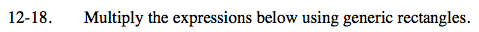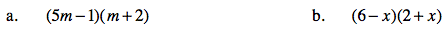Make your generic rectangleand label the dimensions.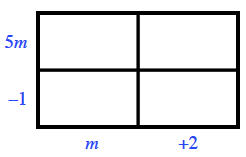Find the area of each of the sections, then add the areas together to get your answer.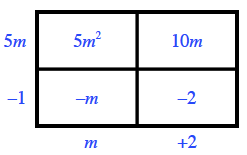5m2 + 9m − 2

See the help for part (a).

12 + 4xx2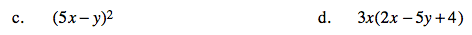See the help for part (a).

See the help for part (a).